• 实验研究了电光相位调制中剩余幅度调制以及它的变化引起的碘稳频532 nm光频标的频率漂移。观测了电光晶体温度与剩余幅度调制和光频标锁定后激光频率之间的变化关系。研究了利用主动电压反馈抑制剩余幅度调制的实际...
• 频率调制(FM)光谱技术中由于激光偏振态变化产生的残余幅度调制(RAM)使其在微量气体检测中的应用受到极大的限制。理论上详细分析了这一过程产生的原因，获得了存在RAM时FM光谱线型的表达式，同时给出N.C.Wong和J.L....
• 三维幅度调制解调技术研究，张长清，，5G系统是一个万物互联的高传输速率和高传输数据量的移动通信网络，需要有高速率基带数字调制解调支撑。传统的高阶QAM方式对传输�
• 目前的模拟调光和脉冲宽度调制(PWM)调光方式都不能解决这一问题,研究发现平均电流影响光通量,峰值电流影响色温,因此提出了一种PWM 和脉冲幅度调制(PAM)调光技术,在保持驱动电流脉冲平均值不变的情况下,调节电流脉冲...
• 36mW2抽头40Gb/s四级脉冲幅度调制发送，王阳，盖伟新，基于SMIC 65-nm CMOS工艺设计了一种40Gb/s低功耗四级脉冲幅度调制(PAM4)发送。设计中的预加重抽头只在输出信号电平转换后紧跟的单位时间集成电路设计
• 研究不同的模拟幅度调制器以及相干解调器模型并且探究其抗噪声性能以及不同模块对于信号的影响。 (2)研究方法 通过构建三种调制方式的相干解调模型，对三种方式的相干解调时的抗干扰性能进行理论分析后，用Matlab...
模拟幅度调制相干解调系统抗噪声性能仿真分析
文章目录模拟幅度调制相干解调系统抗噪声性能仿真分析1、引言(1)研究目的(2)研究方法(3)主要内容2、系统模型（1）常规调幅调制（AM）1.模型建立2.时域分析3.频谱分析（2）抑制载波双边带调幅（DSB-SC）1.模型建立2.时域分析3.频谱分析（3）单边带调制（SSB）1.模型建立2.时域分析3.频谱分析3、抗干扰性能理论分析引言（1）常规调幅调制（AM）（2）抑制载波双边带调幅（DSB-SC）（3）单边带调制（SSB）（4）总结归纳4、仿真代码与结果分析（1）仿真设计：（2）仿真代码：AMDSB-SCSSB（3） 运行截图：AMDSB-SCSSB滤波器设计：抗噪声分析：5、总结6、参考文献
1、引言
要求
(1)研究目的
研究不同的模拟幅度调制器以及相干解调器模型并且探究其抗噪声性能以及不同模块对于信号的影响。
(2)研究方法
通过构建三种调制方式的相干解调模型，对三种方式的相干解调时的抗干扰性能进行理论分析后，用Matlab对于模型进行仿真，观察仿真结果，并与理论结果进行比较分析，最后得出结论。
(3)主要内容

构建AM、DSB-SC、SSB三种模拟幅度调制系统的相干解调模型。
介绍并且分析比较AM、DSB-SC、SSB三种模拟幅度调制系统的原理和对于白噪声的影响。
对三种系统进行Matlab仿真，与理论值进行比较得出结论。

2、系统模型
要求：
（1）给出模型图，以及时域频谱分析等。
（2）所有图都要有编号和标题，要给出图的解释。
（3）所有公式都要有编号，要对公式中第一次出现的符号进行解释。
（1）常规调幅调制（AM）
1.模型建立
AM的调制模型如图1-1所示，显然有：
$s_{AM}(t) = A_c[1+m(t)]cos2\pi f_ct \tag{1.1}$
进一步我们定义调幅指数：
$\beta_{AM}=max|m(t)|\tag{1.2}$图1-1 AM调制器模型
那么我们可以得到抗干扰相干解调模型：图1-2 AM调制器抗干扰相干解调模型
2.时域分析
由公式(1.1)可知调制信号的时域为：
$s_{AM}(t) =A_c[1+m(t)]\text{cos}2\pi f_ct \tag{1.1}$
所以可以得到调制信号的频域为：
$S_{AM}(f) = \frac{A_c}2[\delta(f-f_c)+\delta(f+f_c)]+\frac{A_c}2[M(f-f_c)+M(f+f_c)]\tag{1.3}$
在进行解调之前，我们先通过理想的带通滤波器滤除信号频段外的噪音得到$r(t) = s_{AM}(t) + n(t)$，然后再使用相干解调，与$\text{cos}2\pi f_ct$相乘得到$s_d(t)$，最后经过理想低通滤波器得到输出信号$s_o(t)$。
信号时域分析为，
$s_{AM}(t) = A_c[1+m(t)]\text{cos} 2\pi f_ct\tag{1.1}$
$s_d(t) = \frac12 A_c[1+m(t)][1 + \text{cos} 4\pi f_ct]\tag{1.4}$
$s_o(t) = \frac12 A_cm(t)\tag{1.5}$
噪声时域分析为，
$n(t) = n_c(t)\text{cos} 2\pi f_ct-n_s(t)\text{sin} 2\pi f_ct\tag{1.6}$
$n_d(t) = \frac12 n_c(t) + \frac12n_c(t)\text{cos}4\pi f_ct - \frac12n_s(t)\text{sin}4\pi f_ct\tag{1.7}$
$n_o(t) = \frac12 n_c(t)\tag{1.8}$
3.频谱分析
频域示意图为：
AM调制器：图1-3 AM调制器示意图
AM相干解调器：图1-4 AM相干解调器示意图
（2）抑制载波双边带调幅（DSB-SC）
1.模型建立
DSB-SC的调制模型如图2-1所示，显然有：
​假设双边带抑制载波调幅信号$s(t)$是利用均值为零的模拟基带信号$m(t)$与正弦载波$c(t)$相乘得到：
$s_{DSB}(t) = m(t)\cdot c(t) = m(t)A_c\text{cos}2\pi f_ct \tag{2.1}$
$S_{DSB}(f) = \frac{A_c}2[M(f-f_c)+M(f+f_c)]\tag{2.2}$图2-1 DSB-SC调制器示意图图2-2 DSB-SC抗干扰相干解调器示意图
2.时域分析
在解调前我们先通过理想带通滤波器滤除信号频段以外的噪音信号得到$r(t) = s(t) + n(t)$，再使用相干解调，与$\text{cos}2\pi f_ct$相乘得到$s_d(t)$，通过理想的低通滤波器后，得到输出信号$s_o(t)$。
信号的时域为，
$s(t) = A_cm(t)\text{cos} 2\pi f_ct\tag{2.3}$
$s_d(t) = \frac12 A_cm(t)[1 + \text{cos} 4\pi f_ct]\tag{2.4}$
$s_o(t) = \frac12 A_cm(t)\tag{2.5}$
噪声的时域为，
$n_i(t) = n_c(t)\text{cos} 2\pi f_ct-n_s(t)\text{sin} 2\pi f_ct\tag{2.6}$
$n_d(t) = \frac12 n_c(t) + \frac12n_c(t)\text{cos}4\pi f_ct - \frac12n_s(t)\text{sin}4\pi f_ct\tag{2.7}$
$n_o(t) = \frac12 n_c(t)\tag{2.8}$
3.频谱分析图2-3 DSB-SC调制器频谱示意图
（3）单边带调制（SSB）
1.模型建立
​		AM调制和DSB-SC调制都属于双边带调制，双边带调制的频谱有两个对称的边带，使其带宽是基带信号带宽的2倍。为了节约信号传输中的对信道带宽的占用，可以去除其中一个边带来传输，这种方式就是单边带（SSB）调制：

上单边带信号：
$s_{USSB}(t) = \frac{A_c}2m(t)\text{cos} 2\pi f_ct - \frac{A_c}2\hat m(t)\text{sin} 2\pi f_ct\tag{3.1}$
$S_{USSB}(t) = \frac14[M(f-f_c)+M(f+f_c)-M(f+f_c)\cdot sgn(f+f_c)\\+M(f-f_c)\cdot sgn(f-f_c)]\tag{3.2}$

下单边带信号：
$s_{LSSB}(t) = \frac{A_c}2m(t)\text{cos} 2\pi f_ct + \frac{A_c}2\hat m(t)\text{sin} 2\pi f_ct\tag{3.3}$
$S_{LSSB}(t) = \frac14[M(f-f_c)+M(f+f_c)+M(f+f_c)\cdot sgn(f+f_c)\\-M(f-f_c)\cdot sgn(f-f_c)]\tag{3.4}$

​        解调前先通过理想带通滤波器滤除信号频段外的噪音信号得到$r(t) = s(t) + n(t)$，再使用相干解调，与$\text{cos}2\pi f_ct$相乘得到$s_d(t)$，经过理想低通滤波器并做数值一定处理得到输出信号$s_o(t)$。图3-1 SSB相移法产生单边带信号模型图3-2 SSB调制器模型图3-3 SSB抗干扰相干解调示意图
2.时域分析
信号的时域为，
$s(t) = \frac{A_c}2m(t)\text{cos} 2\pi f_ct\mp \frac{A_c}2\hat m(t)\text{sin} 2\pi f_ct\tag{3.5}$
$s(t) = \frac{A_c}4m(t)+ \frac{A_c}4m(t)\text{cos} 4\pi f_ct\mp \frac{A_c}4\hat m(t)\text{sin} 4\pi f_ct\tag{3.6}$
$s_o(t) = \frac14 A_cm(t)\tag{3.7}$
噪声的时域为，
$n(t) = n_c(t)\text{cos} 2\pi f_ct-n_s(t)\text{sin} 2\pi f_ct\tag{3.8}$
$n_d(t) = \frac12 n_c(t) + \frac12n_c(t)\text{cos}4\pi f_ct - \frac12n_s(t)\text{sin}4\pi f_ct\tag{3.9}$
$n_o(t) = \frac12 n_c(t)\tag{3.10}$
3.频谱分析
以上边带为例进行频谱分析：图3-4 SSB频域分析示意图
3、抗干扰性能理论分析
要求：给出理论分析的过程以及结果
引言
通过前面的分析可知，我们可以根据图3-3 SSB抗干扰相干解调示意图中的$(S/N)_{in}和(S/N)_{out}$以及两者的比值$G = \frac{(S/N)_{out}}{(S/N)_{in}}$来对三种调制方式的抗干扰性能进行分析。
​		不妨假设原信号$m(t)$的功率为$P_m$，带宽为$B$，输入白噪音的双边带功率为$\frac{n_0}2$。
那么我们可以得到以下内容：
$S_{in} = \langle s^2(t)\rangle \tag{4.1}$
$N_{in} = E[n^2(t)]\tag{4.2}$
$S_{out} = \langle s_o^2(t)\rangle\tag{4.3}$
$N_{out} = E[n_o^2(t)]\tag{4.4}$
有了以上结论，我们就可以分别对三种情况进行分析。
（1）常规调幅调制（AM）
​		将(1.3)~(1.8)​代入​(4.1)~(4.4)可得：
$S_{in} = \langle s^2(t)\rangle = \frac{A_c^2}2[1+P_m] \tag{4.5}$
$N_{in} = E[n^2(t)] = 2n_0B \tag{4.6}$
$S_{out} = \langle s_o^2(t)\rangle = \frac{A_c^2P_m}4 \tag{4.7}$
$N_{out} = E[n_o^2(t)] = \frac{n_0B}2 = \frac{N_{in}}4 \tag{4.8}$
​		然后可以推出AM输入和输出的信噪比和相干解调的增益：
$(S/N)_{in} = \frac{S_{in}}{N_{in}} = \frac{A_c^2[1+P_m]}{4n_0B} \tag{4.9}$
$(S/N)_{out} = \frac{S_{out}}{N_{out}} = \frac{A_c^2P_m}{2n_0B} \tag{4.10}$
$G_{AM} = \frac{(S/N)_{out}}{(S/N)_{in}} = \frac{2P_m}{1+P_m} \tag{4.11}$
（2）抑制载波双边带调幅（DSB-SC）
​		将(2.3)~(2.8)代入(4.1)~(4.4)可得：
$S_{in} = \langle s^2(t)\rangle = \frac{A_c^2P_m}2 \tag{4.12}$
$N_{in} = E[n^2(t)] = 2n_0B \tag{4.13}$
$S_{out} = \langle s_o^2(t)\rangle = \frac{A_c^2P_m}4 = \frac{S_{in}}2 \tag{4.14}$
$N_{out} = E[n_o^2(t)] = \frac{n_0B}2 = \frac{N_{in}}4 \tag{4.15}$
​		然后可以推出DSB-SC输入和输出的信噪比和相干解调的增益：
$(S/N)_{in} = \frac{S_{in}}{N_{in}} = \frac{A_c^2P_m}{4n_0B} \tag{4.16}$
$(S/N)_{out} = \frac{S_{out}}{N_{out}} = \frac{A_c^2P_m}{2n_0B} \tag{4.17}$
$G_{DSB-SC} = \frac{(S/N)_{out}}{(S/N)_{in}} = 2 \tag{4.18}$
（3）单边带调制（SSB）
如果能将$s(t)$的能量统一，就会便于比较抗噪声性能，将(3.1)~(3.3)的$s(t)$乘以$\sqrt 2$
​		将(3.5)~(3.10)代入(4.1)~(4.4)中，可得：
$S_{in} = \langle s^2(t)\rangle = \frac{A_c^2P_M}2 \tag{4.19}$
$N_{in} = E[n^2(t)] = n_0B \tag{4.20}$
$S_{out} = \langle s_o^2(t)\rangle = \frac{A_c^2P_M}8 = \frac{S_{in}}4 \tag{4.21}$
$N_{out} = E[n_o^2(t)] = \frac{n_0B}4 = \frac{N_{in}}4 \tag{4.22}$
​		然后可以推出SSB输入和输出的信噪比和相干解调的增益：
$(S/N)_{in} = \frac{S_{in}}{N_{in}} = \frac{A_c^2P_M}{2n_0B} \tag{4.23}$
$(S/N)_{out} = \frac{S_{out}}{N_{out}} = \frac{A_c^2P_M}{2n_0B} \tag{4.24}$
$G_{SSB} = \frac{(S/N)_{out}}{(S/N)_{in}} = 1 \tag{4.25}$
（4）总结归纳$表4.1—抗噪声性能比较$
​		由上表可以得出结论，对于信噪比增益G有：
$G_{DSB} = 2,G_{SSB} = 1,G_{AM} = \frac{2P_M}{1+P_M}$
所以我们可知DSB-SC调制在相干解调后的信噪比是之前的两倍，但输出的信噪比DSB-SC与SSB都是$\frac{A_c^2P_M}{2n_0B}$，所以用DSB-SC还是SSB调制方式输出信噪比相等。
4、仿真代码与结果分析
要求：给出仿真模型、关键的MATLAB语句、仿真结果，以及对于结果的分析，特别是跟理论结果的比较。
（1）仿真设计：
根据前面三种模型进行仿真设计，然后加入对应的噪声进行分析。
（2）仿真代码：
AM
%参数设置
T_start=0;%开始时间
T_stop=5;%截止时间
T=T_stop-T_start;%仿真持续时间
f_sample=1000; % 采样频率
T_sample=1/f_sample;%采样间隔
N_sample=T/T_sample;% 采样点数
f_res=f_sample/N_sample;%频率分辨率
f_max=f_res*N_sample/2;%最大频率
power_dB = -60;%噪声增益
%初始信号设置
Amax=1;%幅度
Am = 0.5;%调制系数
fm=10;%fm频率
fc=100;%fc频率
phrase1=0;%初始相位
n=0:N_sample-1;
%输入信号m(t)时域、频域
mt=Am*Amax*cos(2*pi*fm*n*T_sample+phrase1);
Mf = abs(fft(mt));
Mf_rearrange=[Mf(N_sample/2+1:N_sample-1),Mf(1:N_sample/2)];
%s(t)
ct=Amax*cos(2*pi*fc*n*T_sample+phrase1);
sAMt = (mt+1).*ct;
SAMf = abs(fft(sAMt));
SAMf_rearrange=[SAMf(N_sample/2+1:N_sample-1),SAMf(1:N_sample/2)];
%sd(t)
rt = conv(sAMt,BandPF,'same');
N_sample2 = length(rt(:));%通过DaiTLB后采样点数
if mod(N_sample2,2)~=0%长度化为偶数
rt = rt(1:N_sample2-1);
N_sample2 = N_sample2 -1;
end
n2 = 0:N_sample2-1;
f_res2=f_sample/N_sample2;%通过BPF后频率分辨率
f_max2=f_res2*N_sample2/2;%通过BPF最大频率
m2t = Amax*cos(2*pi*fc*n2*T_sample+phrase1);
sdt = rt.*m2t;
Sdf = abs(fft(sdt));
Sdf_rearrange=[Sdf(N_sample2/2+1:N_sample2-1),Sdf(1:N_sample2/2)];
%yout(t)
youtt = conv(sdt,LowPF,'same');
N_sample3 = length(yot(:));
if mod(N_sample3,2)~=0
youtt = yot(1:N_sample3-1);
N_sample3 = N_sample3 -1;
end
n3 = 0:N_sample3-1;
f_res3=f_sample/N_sample3;
f_max3=f_res3*N_sample3/2;
Youtf = abs(fft(yot));
Youtf_rearrange=[Youtf(N_sample3/2+1:N_sample3-1),Youtf(1:N_sample3/2)];
youtt = youtt-mean(youtt);
nw = wgn(1,N_sample,power_dB);
Noise_w=abs(fft(nw)).^2*T_sample/T/f_sample;
Nw_rearrange=[Noise_w(N_sample/2+1:N_sample-1),Noise_w(1:N_sample/2)];
ni = conv(nw,BandPF,'same');
Noise_i=abs(fft(ni)).^2*T_sample/T/f_sample;
Ni_rearrange=[Noise_i(N_sample/2+1:N_sample-1),Noise_i(1:N_sample/2)];
no = conv(ni.*ct,LowPF,'same');
Noise_o=abs(fft(no)).^2*T_sample/T/f_sample;
No_rearrange=[Noise_o(N_sample/2+1:N_sample-1),Noise_o(1:N_sample/2)];
%波形输出
figure(1);
subplot(4,1,1);
plot(n*T_sample,mt);
xlim([0 0.5]);
xlabel('t/s');ylabel('m(t)');title('m(t)的时域波形');
figure(2);
subplot(4,1,1);
plot((-N_sample/2+1:N_sample/2-1)*f_res,Mf_rearrange);
xlim([-20,20]);
xlabel('f/Hz');ylabel('M(f)');title('M(f)的频谱图');
figure(1);
subplot(4,1,2);
plot(n*T_sample,sAMt);
xlim([0 0.5]);
xlabel('t/s');ylabel('sAM(t)');title('sAM(t)的时域波形');
figure(2);
subplot(4,1,2);
plot((-N_sample/2+1:N_sample/2-1)*f_res,SAMf_rearrange);
xlim([-150 150]);
xlabel('f/Hz');ylabel('SAM(f)');title('SAM(f)的频谱图');
figure(1);
subplot(4,1,3);
plot(n2*T_sample,sdt);
xlim([2 2.5]);
xlabel('t/s');ylabel('sd(t)');title('sd(t)的时域波形');
figure(2);
subplot(4,1,3);
plot((-N_sample2/2+1:N_sample2/2-1)*f_res2,Sdf_rearrange);
xlim([-250 250]);
xlabel('f/Hz');ylabel('Sd(f)');title('Sd(f)的频谱图');
figure(1);
subplot(4,1,4);
plot(n3*T_sample,yot);
xlim([2 2.5]);
xlabel('t/s');ylabel('yo(t)');title('yo(t)的时域波形');
figure(2);
subplot(4,1,4);
plot((-N_sample3/2+1:N_sample3/2-1)*f_res3,Yof_rearrange);
xlim([-250 250]);
xlabel('f/Hz');ylabel('Yo(f)');title('Yo(f)的频谱图');
figure(3);
subplot(1,1,1);
plot((-N_sample/2+1:N_sample/2-1)*f_res,Nw_rearrange);
xlabel('f');ylabel('Pnw(f)');title('高斯白噪音功率谱');
figure(4);
subplot(2,1,1);
plot((-N_sample/2+1:N_sample/2-1)*f_res,Ni_rearrange);
xlabel('f');ylabel('Pni(f)');title('in输入噪音功率谱');
subplot(2,1,2);
plot((-N_sample/2+1:N_sample/2-1)*f_res,No_rearrange);
xlabel('f');ylabel('Pno(f)');title('out输出噪音功率谱');
P_nw = sum(Noise_w)/length(Noise_w)*f_sample
P_ni = sum(Noise_i)/length(Noise_i)*f_sample
P_no = sum(Noise_o)/length(Noise_o)*f_sample
P_rt = sum(rt.^2)/length(rt)
P_yot = sum(yot.^2)/length(yot)
SNR_in = P_rt/P_ni
SNR_out = P_yot/P_no
G_AM = SNR_out/SNR_in

DSB-SC
%参数设置
T_start=0;%开始时间
T_stop=5;%截止时间
T=T_stop-T_start;%仿真持续时间
f_sample=1000; % 采样速率
T_sample=1/f_sample;%采样间隔
N_sample=T/T_sample;% 采样点数
power_dB = -60;%噪声分贝数
f_res=f_sample/N_sample;%频率分辨率
f_max=f_res*N_sample/2;%最大频率

A=1;%幅度
fm=10;%fm频率
fc=100;%fc频率
theta=0;%初始相位
%信号产生
n=0:N_sample-1;
mt=A*cos(2*pi*fm*n*T_sample+theta);
Mf = abs(fft(mt));
Mf_rearrange=[Mf(N_sample/2+1:N_sample-1),Mf(1:N_sample/2)];
ct=A*cos(2*pi*fc*n*T_sample+theta);
s_DSBt = mt.*ct;
S_DSBf = abs(fft(s_DSBt));
S_DSBf_rearrange=[S_DSBf(N_sample/2+1:N_sample-1),S_DSBf(1:N_sample/2)];
rt = conv(s_DSBt,BandPF,'same');
N_sample2 = length(rt(:));
if mod(N_sample2,2)~=0
rt = rt(1:N_sample2-1);
N_sample2 = N_sample2 -1;
end
n2 = 0:N_sample2-1;
f_res2=f_sample/N_sample2;
f_max2=f_res2*N_sample2/2;
m2t = A*cos(2*pi*fc*n2*T_sample+theta);
sdt = rt.*m2t;
Sdf = abs(fft(sdt));
Sdf_rearrange=[Sdf(N_sample2/2+1:N_sample2-1),Sdf(1:N_sample2/2)];
%输出信号
yot = conv(sdt,LowPF,'same');
N_sample3 = length(yot(:));
if mod(N_sample3,2)~=0
yot = yot(1:N_sample3-1);
N_sample3 = N_sample3 -1;
end
n3 = 0:N_sample3-1;
f_res3=f_sample/N_sample3;
f_max3=f_res3*N_sample3/2;
Yof = abs(fft(yot));
Yof_rearrange=[Yof(N_sample3/2+1:N_sample3-1),Yof(1:N_sample3/2)];

yot = yot-mean(yot);
nw = wgn(1,N_sample,power_dB);
Noise_w=abs(fft(nw)).^2*T_sample/T/f_sample;
Nw_rearrange=[Noise_w(N_sample/2+1:N_sample-1),Noise_w(1:N_sample/2)];
ni = conv(nw,BandPF,'same');
Noise_i=abs(fft(ni)).^2*T_sample/T/f_sample;
Ni_rearrange=[Noise_i(N_sample/2+1:N_sample-1),Noise_i(1:N_sample/2)];
no = conv(ni.*ct,LowPF,'same');
Noise_o=abs(fft(no)).^2*T_sample/T/f_sample;
No_rearrange=[Noise_o(N_sample/2+1:N_sample-1),Noise_o(1:N_sample/2)];
figure(1);
subplot(4,1,1);
plot(n*T_sample,mt);
xlim([0 0.5]);
xlabel('t/s');
ylabel('m(t)');
title('m(t)的时域波形');
figure(2);
subplot(4,1,1);
plot((-N_sample/2+1:N_sample/2-1)*f_res,Mf_rearrange);
xlim([-20,20]);
xlabel('f/Hz');
ylabel('M(f)');
title('M(f)的频谱图');
figure(1);
subplot(4,1,2);
plot(n*T_sample,s_DSBt);
xlim([0 0.5]);
xlabel('t/s');
ylabel('s(t)');
title('s(t)的时域波形');
figure(2);
subplot(4,1,2);
plot((-N_sample/2+1:N_sample/2-1)*f_res,S_DSBf_rearrange);
xlim([-150 150]);
xlabel('f/Hz');
ylabel('S(f)');
title('S(f)的频谱图');
figure(1);
subplot(4,1,3);
plot(n2*T_sample,sdt);
xlim([2 2.5]);
xlabel('t/s');
ylabel('sd(t)');
title('sd(t)的时域波形');
figure(2);
subplot(4,1,3);
plot((-N_sample2/2+1:N_sample2/2-1)*f_res2,Sdf_rearrange);
xlim([-250 250]);
xlabel('f/Hz');
ylabel('Sd(f)');
title('Sd(f)的频谱图');
figure(1);
subplot(4,1,4);
plot(n3*T_sample,yot);
xlim([2 2.5]);
xlabel('t/s');
ylabel('yo(t)');
title('yo(t)的时域波形');
figure(2);
subplot(4,1,4);
plot((-N_sample3/2+1:N_sample3/2-1)*f_res3,Yof_rearrange);
xlim([-250 250]);
xlabel('f/Hz');
ylabel('Yo(f)');
title('Yo(f)的频谱图');
figure(3);
subplot(2,1,1);
plot((-N_sample/2+1:N_sample/2-1)*f_res,Ni_rearrange);
xlabel('f');ylabel('Pni(f)');title('输入噪音功率谱');
subplot(2,1,2);
plot((-N_sample/2+1:N_sample/2-1)*f_res,No_rearrange);
xlabel('f');ylabel('Pno(f)');title('输出噪音功率谱');
P_nw = sum(Noise_w)/length(Noise_w)*f_sample
P_ni = sum(Noise_i)/length(Noise_i)*f_sample
P_no = sum(Noise_o)/length(Noise_o)*f_sample
P_rt = sum(rt.^2)/length(rt)
P_yot = sum(yot.^2)/length(yot)
SNR_in = P_rt/P_ni
SNR_out = P_yot/P_no
G_DSB = SNR_out/SNR_in

SSB
T_start=0;%开始时间
T_stop=5;%截止时间
T=T_stop-T_start;%仿真持续时间
f_sample=1000; % 采样速率
T_sample=1/f_sample;%采样间隔
N_sample=T/T_sample;% 采样点数
power_dB = -60;%噪声分贝数
f_res=f_sample/N_sample;%频率分辨率
f_max=f_res*N_sample/2;%最大频率
A=1;%幅度
fm=10;%fm频率
fc=100;%fc频率
theta=0;%初始相位
%产生信号
n=0:N_sample-1;
mt=A*cos(2*pi*fm*n*T_sample+theta);
Mf = abs(fft(mt));
Mf_rearrange=[Mf(N_sample/2+1:N_sample-1),Mf(1:N_sample/2)];
ct=A*cos(2*pi*fc*n*T_sample+theta);
c2t=A*sin(2*pi*fc*n*T_sample+theta);
m2t=hilbert(mt);
m2t = imag(m2t);
s_SSBt = 2^0.5/2*(mt.*ct - m2t.*c2t);
S_SSBf = abs(fft(s_SSBt));
S_SSBf_rearrange=[S_SSBf(N_sample/2+1:N_sample-1),S_SSBf(1:N_sample/2)];

rt = conv(s_SSBt,BandPF2,'same');
N_sample2 = length(rt(:));
if mod(N_sample2,2)~=0
rt = rt(1:N_sample2-1);
N_sample2 = N_sample2 -1;
end
n2 = 0:N_sample2-1;
f_res2=f_sample/N_sample2;
f_max2=f_res2*N_sample2/2;
m2t = A*cos(2*pi*fc*n2*T_sample+theta);
sdt = rt.*m2t;
Sdf = abs(fft(sdt));
Sdf_rearrange=[Sdf(N_sample2/2+1:N_sample2-1),Sdf(1:N_sample2/2)];
%输出信号
yot = conv(sdt,LowPF,'same');
N_sample3 = length(yot(:));
if mod(N_sample3,2)~=0
yot = yot(1:N_sample3-1);
N_sample3 = N_sample3 -1;
end
n3 = 0:N_sample3-1;
f_res3=f_sample/N_sample3;
f_max3=f_res3*N_sample3/2;
Yof = abs(fft(yot));
Yof_rearrange=[Yof(N_sample3/2+1:N_sample3-1),Yof(1:N_sample3/2)];

yot = yot-mean(yot);

%噪声
nw = wgn(1,N_sample,power_dB);
Noise_w=abs(fft(nw)).^2*T_sample/T/f_sample;
Nw_rearrange=[Noise_w(N_sample/2+1:N_sample-1),Noise_w(1:N_sample/2)];
ni = conv(nw,BandPF2,'same');
Noise_i=abs(fft(ni)).^2*T_sample/T/f_sample;
Ni_rearrange=[Noise_i(N_sample/2+1:N_sample-1),Noise_i(1:N_sample/2)];
no = conv(ni.*ct,LowPF,'same');
Noise_o=abs(fft(no)).^2*T_sample/T/f_sample;
No_rearrange=[Noise_o(N_sample/2+1:N_sample-1),Noise_o(1:N_sample/2)];

figure(1);
subplot(4,1,1);
plot(n*T_sample,mt);
xlim([0 0.5]);
xlabel('t/s');
ylabel('m(t)');
title('m(t)的时域波形');
figure(2);
subplot(4,1,1);
plot((-N_sample/2+1:N_sample/2-1)*f_res,Mf_rearrange);
xlim([-20,20]);
xlabel('f/Hz');
ylabel('M(f)');
title('M(f)的频谱图');
figure(1);
subplot(4,1,2);
plot(n*T_sample,s_SSBt);
xlim([0 0.5]);
xlabel('t/s');
ylabel('s(t)');
title('s(t)的时域波形');
figure(2);
subplot(4,1,2);
plot((-N_sample/2+1:N_sample/2-1)*f_res,S_SSBf_rearrange);
xlim([-150 150]);
xlabel('f/Hz');
ylabel('S(f)');
title('S(f)的频谱图');
figure(1);
subplot(4,1,3);
plot(n2*T_sample,sdt);
xlim([2 2.5]);
xlabel('t/s');
ylabel('sd(t)');
title('sd(t)的时域波形');
figure(2);
subplot(4,1,3);
plot((-N_sample2/2+1:N_sample2/2-1)*f_res2,Sdf_rearrange);
xlim([-250 250]);
xlabel('f/Hz');
ylabel('Sd(f)');
title('Sd(f)的频谱图');
figure(1);
subplot(4,1,4);
plot(n3*T_sample,yot);
xlim([2 2.5]);
xlabel('t/s');
ylabel('yo(t)');
title('yo(t)的时域波形');
figure(2);
subplot(4,1,4);
plot((-N_sample3/2+1:N_sample3/2-1)*f_res3,Yof_rearrange);
xlim([-250 250]);
xlabel('f/Hz');
ylabel('Yo(f)');
title('Yo(f)的频谱图');
figure(3);
subplot(2,1,1);
plot((-N_sample/2+1:N_sample/2-1)*f_res,Ni_rearrange);
xlabel('f');ylabel('Pni(f)');title('输入噪音功率谱');
subplot(2,1,2);
plot((-N_sample/2+1:N_sample/2-1)*f_res,No_rearrange);
xlabel('f');ylabel('Pno(f)');title('输出噪音功率谱');
P_nw = sum(Noise_w)/length(Noise_w)*f_sample
P_ni = sum(Noise_i)/length(Noise_i)*f_sample
P_no = sum(Noise_o)/length(Noise_o)*f_sample
P_rt = sum(rt.^2)/length(rt)
P_yot = sum(yot.^2)/length(yot)
SNR_in = P_rt/P_ni
SNR_out = P_yot/P_no
G_SSB = SNR_out/SNR_in


（3） 运行截图：
AM图4-1 AM时域仿真示意图图4-2 AM频域仿真示意图
DSB-SC图4-3 DSB-SC时域仿真示意图图4-4 DSB-SC频域仿真示意图
SSB图4-5 SSB时域仿真示意图图4-5 SSB频域仿真示意图
滤波器设计：图4-5 BandPF1滤波器图4-6 BandPF2滤波器图4-7 LowPF滤波器
抗噪声分析：图4-8 高斯白噪声功率谱图4-9 AM噪声功率谱图4-10 DSB-SC噪声功率谱图4-11 SSB噪声功率谱

抗噪声分析
AM
DSB-SC
SSB

S_in
0.6062
0.2592
0.2737

N_in
6.2135e-08
6.5561e-08
2.8272e-08

S_out
0.0272
0.1097
0.0581

N_out
1.0038e-08
1.1199e-08
3.2028e-09

SNR_in
9.7564e+06
3.9538e+06
9.6816e+06

SNR_out
2.7108e+06
9.7989e+06
1.8148e+07

G
0.2778
2.4783
1.8745

5、总结
通过分析三种不同的调制解调方式（AM,DSB-SC,SSB）了解调制解调的原理和机制，并且根据模型进行仿真，收益匪浅。
考虑噪声干扰的性能，对比$SNR_{out}$可以得知，DSB-SC和SSB相同，抗噪声性能相当。
进行仿真之后，发现理论和仿真有一定的误差，误差可能有多种来源但并不影响基本结论。
通过仿真我们学会了怎么分析系统的调制解调的抗噪声性能。
6、参考文献
现代通信原理6.1 常规调幅调制(AM)与抑制载波双边带(DSB-SC)调制
现代通信原理6.2：单边带(SSB)调制


展开全文matlab 信号处理
• 若要用幅度调制器和相位调制器分别实现幅度与相位的调制，则电路要复杂和困难得多。信道容量：是指信道极限的传输能力，它常用最大信息速率来表述。传输速率：在数据传输中，定义有三种传输速率：1)数据传输速率指...
用IQ调制器可以方便实现数字调制。大多数的数字调制将数据映射到IQ平面上的若干点，这些点被称为星座点。当信号从一个点移到另一点时，幅度及相位的调制往往同时完成了。若要用幅度调制器和相位调制器分别实现幅度与相位的调制，则电路要复杂和困难得多。信道容量：是指信道极限的传输能力，它常用最大信息速率来表述。传输速率：在数据传输中，定义有三种传输速率：1)数据传输速率指单位时间内传输的数据量。数据量单位可以是比特、字符、码组等，时间单位可以是秒、分、小时。2)数据信号速率如数据传输速率使用比特/秒(bps、bit/s、B/s或b/s)为单位，此时数据传输速率则称作数据信号速率，也称为比特率，记作Rb。它实际上就是前面信息量计算中提到信息传输速率，简称信息速率或传信率、它表示单位时间内系统传送的(或者说通过信道的)信息量。3)码元传输速率又称波特率、传码率或调制速率，记作RB。指单位时间所传送的码元(即脉冲)数目，或者表示信号调制过程中单位时间内调制信号波形的变换次数。单位为波特(Baud或Bd)。(Bd=1/T, T为调制信号周期)
展开全文• 编写幅度调制代码(1)产生一个信息信号(2)产生一个载波信号(3)调制Step3. 添加噪声Step4. 带通滤波Step5. 相干解调(1)经过乘法(2)低通滤波(3) 频谱绘制第一步：Y = fft(X); 将信号X进行傅里...
实现一个基本的AM 或DSB 调制解调系统3.1.2 拓展内容：实现一个SSB 调制解调系统3.2 实验步骤Step1. 打开matlab 新建一个M 文件Step2. 编写幅度调制代码(1)产生一个信息信号(2)产生一个载波信号(3)调制Step3. 添加噪声Step4. 带通滤波Step5. 相干解调(1)经过乘法器(2)低通滤波(3) 频谱绘制第一步：Y = fft(X); 将信号X进行傅里叶变换，点数为信号长度。第二步：f=(0:40000)*fs/40001-fs/2;第三步：plot(f,fftshift(abs(Y))); fftshift是将FFT的直流分量移到频谱中心。4. 实验报告内容及要求(1) 选择AM或DSB中的任何一个写出：实验原理框图，实验结果及分析，程序源代码;(2) 要求画出信号频率为10Hz，载波频率为50Hz,采样率为1000Hz,信噪比为5时，对应框图每一点的波形及频谱图(信息信号，载波信号，已调制信号(0%；50%；100%调制)，通过带通滤波器后的信号，解调后的信号)并附上程序源代码。源程序：2.AM调制解调系统程序代码。% AM调制figure('Name','信号调制过程中波形及其频谱','NumberTitle','off')a0=2;f0=10;fc=50;fs=1000;snr=5;t=[-20:0.001:20];am1=cos(2*pi*f0*t); %信息信号am=a0+am1;t1=cos(2*pi*fc*t); %载波s_am=am.*t1;AM1=fft(am1); T1=fft(t1); S_AM=fft(s_am);f=(0:40000)*fs/40001-fs/2;subplot(3,2,1); plot(t(19801:20200),am1(19801:20200)); title('信息信号波形');subplot(3,2,2); plot(f,fftshift(abs(AM1))); title('信息信号频谱');subplot(3,2,3); plot(t(19801:20200),t1(19801:20200)); title('载波信号');subplot(3,2,4); plot(f,fftshift(abs(T1))); title('载波信号频谱');subplot(3,2,5); plot(t(19801:20200),s_am(19801:20200)); title('已调信号');subplot(3,2,6); plot(f,fftshift(abs(S_AM))); title('已调信号频谱');%产生噪声figure('Name','添加噪声及带通滤波过程波形及其频谱','NumberTitle','off');y=awgn(s_am,snr);a=[35,65];b=[30,70];Wp=a/(fs/2);Ws=b/(fs/2);Rp=3; Rs=15;[N,Wn]= Buttord(Wp,Ws,Rp,Rs) ;[B,A]=Butter(N,Wn,'bandpass');q=filtfilt(B,A,y);Q=fft(q);Y=fft(y);subplot(2,2,1);plot(t(19801:20200),y(19801:20200));title('添加噪声后信号波形');subplot(2,2,2);plot(f,fftshift(abs(Y)));title('添加噪声后信号频谱');subplot(2,2,3);plot(t(19801:20200),q(19801:20200));title('带通滤波后信号波形');subplot(2,2,4); plot(f,fftshift(abs(Q)));title('带通滤波后信号频谱');%解调figure('Name','相干解调所得波形及其频谱','NumberTitle','off');ss_am=q.*t1;Wp=15/(fs/2);Ws=40/(fs/2);Rp=3; Rs=20;[N,Wn]= Buttord(Wp,Ws,Rp,Rs) ;[B,A]=Butter(N,Wn,'low');m0=filtfilt(B,A,ss_am);M0=fft(m0);subplot(2,1,1);plot(t(19801:20200),m0(19801:20200));title('解调信号');subplot(2,1,2); plot(f,fftshift(abs(M0)));title('解调信号频谱');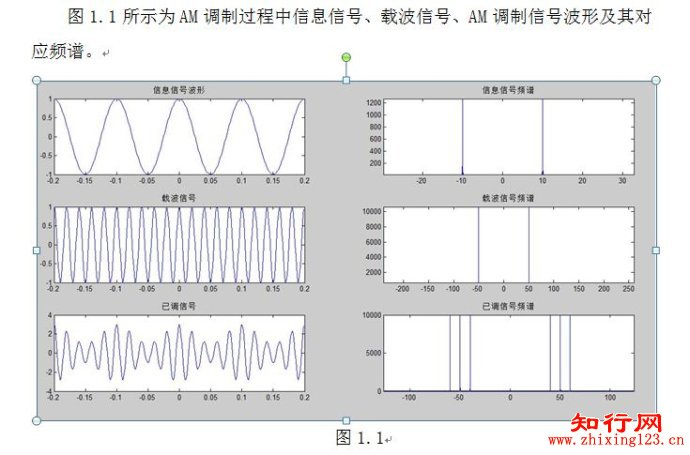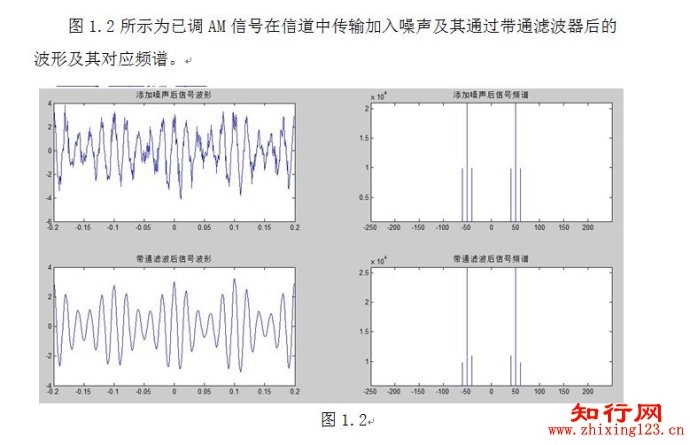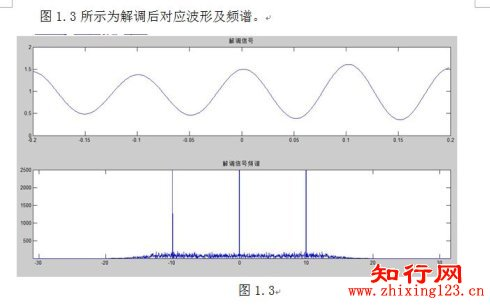二、SSB调制解调系统2.SSB调制解调系统程序代码。% SSB调制figure('Name','SSB信号调制过程中波形及其频谱','NumberTitle','off')f0=10;fc=50;fs=1000;snr=5;t=[-20:0.001:20];am=cos(2*pi*f0*t);am1=sin(2*pi*f0*t);t1=cos(2*pi*fc*t);t2=sin(2*pi*fc*t);s_dsb=am.*t1; %DSB信号Wp=55/(fs/2);Ws=45/(fs/2);Rp=3; Rs=20; %高通滤波器[N,Wn]= Buttord(Wp,Ws,Rp,Rs) ;[B,A]=Butter(N,Wn,'high');ssb1=filtfilt(B,A,s_dsb); %上边带Wp=45/(fs/2);Ws=55/(fs/2);Rp=3; Rs=20; %低通滤波器[N,Wn]= Buttord(Wp,Ws,Rp,Rs) ;[B,A]=Butter(N,Wn,'low');ssb2=filtfilt(B,A,s_dsb); %下边带AM=fft(am); T1=fft(t1); SSB1=fft(ssb1);SSB2=fft(ssb2);f=(0:40000)*fs/40001-fs/2;subplot(3,2,1); plot(t(19801:20200),am(19801:20200)); title('信息信号波形');subplot(3,2,2); plot(f,fftshift(abs(AM))); title('信息信号频谱');subplot(3,2,3); plot(t(19801:20200),t1(19801:20200)); title('载波信号');subplot(3,2,4); plot(f,fftshift(abs(T1))); title('载波信号频谱');subplot(3,2,5);plot(t(19801:20200),ssb1(19801:20200),':',t(19801:20200),ssb2(19801:20200));title('已调信号(虚线-上边带/实线-下边带)');subplot(3,2,6);plot(f,fftshift(abs(SSB1)),':',f,fftshift(abs(SSB2)));title('SSB调制信号频谱(虚线-上边带/实线-下边带)');legend('上边带','下边带');figure('Name','下边带-添加噪声及带通滤波过程波形及其频谱','NumberTitle','off');%加噪声y=awgn(ssb2,snr); %以下边带为例设计a=[35,65];b=[30,70];Wp=a/(fs/2);Ws=b/(fs/2);Rp=3; Rs=15;[N,Wn]= Buttord(Wp,Ws,Rp,Rs) ;[B,A]=Butter(N,Wn,'bandpass');q=filtfilt(B,A,y);Q=fft(q);Y=fft(y);subplot(2,2,1);plot(t(19851:20050),y(19851:20050));title('添加噪声后信号波形');subplot(2,2,2);plot(f,fftshift(abs(Y)));title('添加噪声后信号频谱');subplot(2,2,3);plot(t(19801:20200),q(19801:20200));title('带通滤波后信号波形');subplot(2,2,4); plot(f,fftshift(abs(Q)));title('带通滤波后信号频谱');%解调figure('Name','下边带-相干解调所得波形及其频谱','NumberTitle','off');s_ssb2=q.*t1;Wp=15/(fs/2);Ws=40/(fs/2);Rp=3; Rs=20;[N,Wn]= Buttord(Wp,Ws,Rp,Rs) ;[B,A]=Butter(N,Wn,'low');m0=filtfilt(B,A,s_ssb2);M0=fft(m0);subplot(2,1,1);plot(t(19801:20200),m0(19801:20200));title('解调信号');subplot(2,1,2); plot(f,fftshift(abs(M0)));title('解调信号频谱');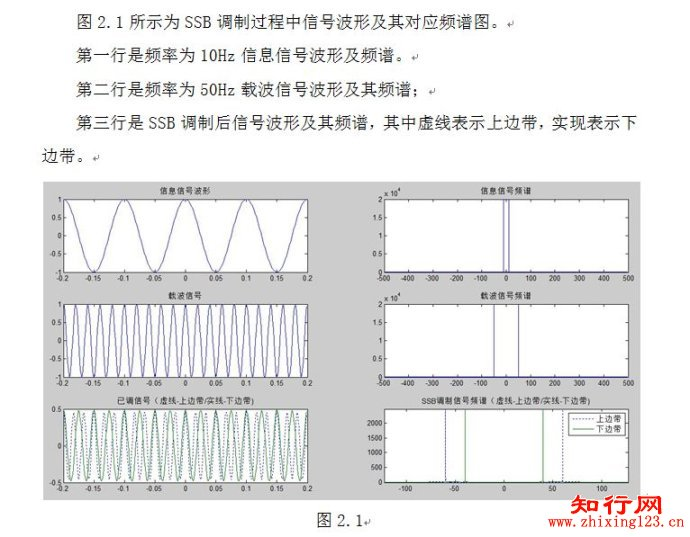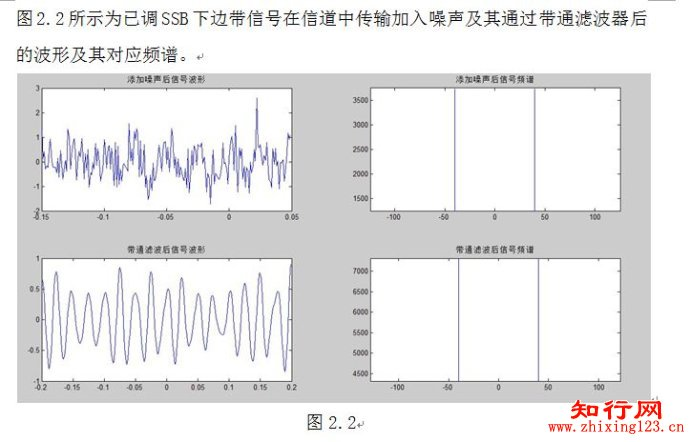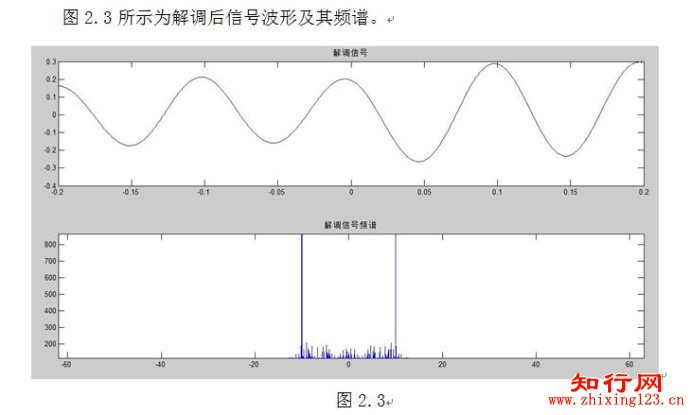展开全文• 幅度调制（线性调制）详解1、基本概念1.1、调制与解调1.2、调制的目的1.3、调制的分类2、幅度调制2.1、基础知识2.2、幅度调制的一般模型2.3、常规调幅 AM(Amplitude Modulation)2.3.1、AM-调幅2.3.2、AM-包络检波...
通信原理系列文章：
通信原理之模拟幅度调制（线性调制）
通信原理之模拟角度调制(非线性调制)
通信原理之模拟调制系统信号的抗造性能
通信原理之数字调制原理
通信原理之模拟幅度调制（线性调制）详解1、基本概念1.1、调制与解调1.2、调制的目的1.3、调制的分类2、幅度调制2.1、基础知识2.2、幅度调制的一般模型2.3、常规调幅 AM(Amplitude Modulation)2.3.1、AM-调幅2.3.2、AM-包络检波器2.3.3、调幅系数（调幅度）m2.3.4、AM调制效率（功率利用率）2.4、双边带调制（DSB-SC）2.4.1、双边带调制（DSB-SC）2.4.2、DSB相干解调2.5、单边带调制 SSB2.6、残留边带调制 VSB
1、基本概念
1.1、调制与解调
调制，即把消息信号寄托在载波的某个参数上，形成已调信号。已调信号的某个参量，如幅度可以反映消息的变化规律。消息信号可分为调制信号和基带信号，载波可为正弦波或脉冲序列。解调，是调制的逆过程，从已调信号中恢复消息信号。
通俗来讲，消息就相当于货物，车相当于载波。调制相当于装载，解调相当于卸载。
1.2、调制的目的
（1）无线通信对天线尺寸减小的需求
在无线通信中，需要通过天线将信号辐射到空间，根据天线理论
$h≥\frac{\lambda}{10}$
其中，h为天线的几何尺寸，$\lambda$ 为发射信号的波长。又信号的波长和频率有以下关系:
$\lambda=\frac{c}{f}$
其中 c为光速 $3x10^8m/s$。故，若发射信号的频率较低时，如 3kHz，则需要大约 10000m长的天线，显然这是很难实现的。若减小天线尺寸，就需要提高发射信号的频率，通过调制将低频信号搬到高频载波上。如GSM 900MHz 手机的天线只有几里面完全内置在手机内，外观看不到天线。
（2）一条信道中同时传输多路信号
因为调制的实质是频谱搬移，故可以通过调制将多路信号搬移到各自的子信道中，实现多路复用。
（3）利用电话线将PC机接入Internet
利用电话线将PC机接入Internet时，需要通过一个“猫”(Modem) 进行"翻译”（模/数信号转换）。
综上，可知调制的目的有以下几点：

匹配信道特性，减小天线尺寸，提高辐射效率；
进行频谱搬移，实现信道的多路复用，提高信道利用率；
扩展信号带宽，提高系统抗干扰能力；
实现带宽与信噪比的互换，即有效性和可靠性的互换；

1.3、调制的分类
按调制信号m(t)的类型，可分为m(t)为模拟信号时称为模拟调制，为数字时称为数字调制。
按已调信号的频谱结构是否保留了原来消息信号的频谱模样，可分为 线性调制和非线性调制。
按载波类型进行分类，若采用正弦型载波则称为连续波调制，若采用脉冲串则称为脉冲调制。
按正弦载波的受调参量，可分为 幅度调制、频率调制和相位调制。
模拟调制是用模拟的消息信号去控制正弦载波的幅度、频率和相位的调制方式。
2、幅度调制
2.1、基础知识
$\begin{matrix} 时域卷积 & f_1(t)*f_2(t)\Leftrightarrow F_1(w)F_2(w)\\ \\ 频域卷积 & f_1(t)f_2(t)\Leftrightarrow \frac{1}{2\pi }F_1(w)*F_2(w)\\ \\ 频移特性 & f(t)e^{\pm jw_ct}\Leftrightarrow F(w \mp w_c)\\ \\ 调制定理 & f(t)cosw_ct \Leftrightarrow \frac{1}{2}[F(w-w_c)+F(w+w_c)]\\ \\ 欧拉公式 & cosw_ct=\frac{1}{2}(e^{jw_ct}+d^{-jw_ct}) \Leftrightarrow \pi [\delta (w-w_c)+\delta (w+w_c)]\\ \\ 单位冲击响应 & 1 \Leftrightarrow 2 \pi \delta(w) \\ \\ 傅里叶变换对及其尺度变换 & 2\pi \delta (w)\Leftrightarrow\delta(f)\\ \\ \end{matrix}$
2.2、幅度调制的一般模型
幅度调制，即通过消息信号控制正弦载波的幅度。其中，消息信号 m(t) 也称基带调制信号，$cosw_ct$ 为载波， h(t) 为 滤波器的冲击响应，$s_m(t)$ 是幅度已调信号。则幅度调制的时域表达式为
$s_m(t)=[m(t)cosw_ct]*h(t)$
频域表达式为
$S_m(w)=\frac{1}{2}[M(w+w_c)+M(w-w_c)]H(w)$
只要适当选择滤波器的特性，则可得到以下几种幅度调制，AM、DSB、SSB、VSB，他们之间的关系如下图所示。若m(t) 是确知信号，可用傅氏变换 $M(w)$ 进行谱分析，若是随机信号，则需要通过功率谱 $H(w)$ 来描述。2.3、常规调幅 AM(Amplitude Modulation)
2.3.1、AM-调幅
对于均值为0的消息信号m(t)，外加直流偏置 $A_0$，得到 $A_0+m(t)$，然后与载波相乘即可得到AM信号。对应调制器的模型为当满足 信息信号的最大值不超过直流偏置 时，即 $|m(t)|_{max} ≤ A_0$ 时，AM波的包络正比于信息信号的变换规律。AM的时间表达式分为 载波项+边带项，可记为
$s_AM(t)=[A_0+m(t)]cosw_ct=A_0cosw_ct+m(t)cosw_ct$
注意，基带信号中的 $A_0$ 为直流分量，调制后的 $A_0$ 为载波幅度。对时间表达式进行傅里叶变换，可以得到AM信号的频谱
$A_{AM}(w)=\pi A_0[\delta (w+w_c)+\delta (w-w_c)]+\frac{1}{2}[M(w+w_c)+M(w-w_c)]$
相应的AM波形和时间频谱，如下所示可见，

时域波形相乘（$[A_0+m(t)]cosw_ct$）对应频域频谱卷积。
从频谱上看，由载波分量，上边带（USB）、下边带（LSB）三部分组成。下边带是上边带的镜像。可见 AM是含有载波分量的双边带信号。传输带宽是基带信号带宽的两倍$B_{AM}=2f_H$ , $B_基=\frac{w_H}{2\pi}=f_H$ 。且已调信号中载波分量是基带信号中的直流分量的搬移，边带谱是消息信号谱的搬移，搬移过程中谱的结构没有发生变化。故幅度调制又称线性调制。
从波形来看，波形的包络正比于 m(t)，这个特点使其可以采用简单的包罗检波进行解调。
因为接收机简单，AM被广泛应用于中短波调幅广播。

2.3.2、AM-包络检波器
AM-包络检波器，可以检出AM信号($S_{AM}(t)$)的包络，隔去直流$A_0$即可还原原来的消息信号$m(t)$。通常由整流器和低通滤波器组成。AM-包络检波器的特点是，简单，不需要相干载波（非相干解调）。但是，它在小信噪比时有门限效应。
2.3.3、调幅系数（调幅度）m
调幅系数，不仅用来反映调制信号 m(t) 改变载波幅度的程度，还涉及 AM 的 功率分配 和 调制效率。可用以下公式来表示：
$m=\frac{|m(t)|_{max}}{A_0}$由上图可见，当 调幅系统 m<1 时，为 正常调幅状态； m=1 时为 临界状态, 称之为 满调幅（100%调幅）；当 m>1 时，将会发生包络失真，称之为 过调幅 。
2.3.4、AM调制效率（功率利用率）
由 AM 信号的表达式
$S_{AM}(t)=A_0cosw_ct+m(t)cosw_ct$，
计算其均方值，可得 AM 信号的功率
$P_{AM}=\frac{A_0^2}{2}+\frac{\overline{m^2(t)}}{2}=P_c+P_s$
其中，$\frac{A_0^2}{2}$ 是载波功率，$\frac{\overline{m^2(t)}}{2}$ 是边带功率。调制效率可以表示为：
$\eta_{AM}=\frac{P_s}{P_{AM}}=\frac{\overline{m^2(t)}}{A_0^2+\overline{m^2(t)}}$
因为 $|m(t)|_{max} ≤ A_0$，故 $\overline{m^2(t)} ≤ A_0^2$, 故调制效率 $\eta_{AM}≤50$% 。这意味着，分配给有用信号，即边带信号的功率很低，最多是总功率的 1/2 。因此 AM调制的功率利用率是很低的。
例，设单音正弦信号 $m(t)=A_mcosw_mt$，其最大值是 $|m(t)|_{max}=A_m$，其均方值 $\overline{m^2(t)}=\frac{A^2_m}{2}$，则其调幅系数为，
$m=\frac{|m(t)|_{max}}{A_0}=\frac{A_m}{A_0}$
其调幅效率为，
$\eta_{AM}=\frac{\overline{m^2(t)}}{A_0^2+\overline{m^2(t)}}=\frac{A^2_m}{2A^2_0+A^2_m}=\frac{m^2}{2+m^2}$
当 m=1 时，调制效率是1/3，调制效率和调制系数的取值和消息信号的波形均有关。当消息信号 m(t) 一定时，调制系数越大，调制效率越高。但在实际中，为了避免过调幅，通常取 $m=0.3\sim 0.6$ 。其原因，主要是载波信号 不含有用信息，但却占用大部分发射功率 $P_c=\frac{A^2_0}{2}$，从而使得 调制效率低。同时，也正是利用这种功率上的浪费，换取了解调的简单，通过包络检波的方法还原消息信号。
2.4、双边带调制（DSB-SC）
2.4.1、双边带调制（DSB-SC）
抑制载波的的双边带调制，Double SideBand Suppressed Carrier（DSB-SC），可以抑制已调信号中的载波分量，等效于去掉基带信号中的直流偏置 $A_0$ ，这种方式称为 抑制载波的的双边带调制，简称双边带调制 DSB。其调制效率可达到 100%，即发射功率都用于有用信号（边带信号），也正因于此，DSB信号的包络不正比于m(t)信号。对应模型为：对应表达式为:
$s_{DSB}=m(t)cosw_ct$
其中 $\overline{m(t)}=0$。进行傅里叶变换后，得到其频谱为
$S_DSB(w)=\frac{1}{2}[M(w+w_c)+M(w-w_c)]$
计算其均方值，得到其调制功率为：
$P_{DSB}=\frac{\overline{m^2(t)}}{2}=P_s$
调制效率为：
$\eta _{DSB}=\frac{P_s}{P_{DSB}}=100$%
DSB 信号的波形与频谱，如下所示：可见，

从频谱上看，DSB信号与AM信号的频谱相似，都有上、下两个边带，带宽都是基带带宽的2倍，$B_{DSB}=B_{AM}=2f_H$，只是没有了载波分量，因此调制效率为100%。
从时域波形上看，DSB信号在调制信号m(t)的零交点处，发生载波反相，即相位突变180度，因此DSB信号的包络不在于m(t)成正比，而AM信号的包络是正比于 m(t)的，不能采用包络检波。
DSB常用于 SSB/VSB 的基础，调频立体声中的差信号调制。

2.4.2、DSB相干解调
解调是调制的逆过程，由前面部分的内容可知，调制过程为：

从时域角度，消息信号m(t)（基带信号）与调制载波 $c(t)=cosw_ct$ 相乘得到已调信号 $s_m(t)$。从频域角度，把消息信号的谱 $M(w)$，从0频处，左、右平移 $\mp w_c$ , 即搬移到正负 $w_c$ 处。而解调是调制的逆过程，则需要将已调信号谱再搬回到0频处，仿照调制过程，将已调信号 $s_m(t)$ 乘以与调制信号c(t) 同频同相的相关载波,则对应频域上，已调信号的频谱左、右平移 $w_c$, 即搬移到了 $\pm 2w_c$ 和 0频处。再经过低通滤波即可还原调制信号 m(t)，这个过程就是相干解调 的设计思想。注意：调制器中的滤波器是带通，而解调器中的滤波器是低通滤波器。相干解调，要求：

载波同步 $c(t)=cosw_ct$，即接收端提供的本地载波与发送端的调制载波通频同相。
故相干解调，也称为 同步检波。它不仅使用与DSB，对所有的线性调制 AM、DSB、SSB、VSB 都适用。
例，某DSB信号 $s_m(t)=m(t)cosw_ct$ 与同步载波相乘，即有

$s_m(t)\cdot c(t)=m(t)cos^2w_ct=m(t)\frac{cos2w_ct+1}{2}=\frac{1}{2}m(t)cos2w_ct+\frac{1}{2}m(t)$
(这里用了倍角公式来化简)经过低通滤波，解调输出 调制信号 $/frac{1}{2}m(t)$。但如若接收端提供的本地载波不相干，如同频不同相，存在载波相位差，则会出现
$s_m(t)\cdot c(t)=m(t)cosw_ct \cdot cos(w_ct+\varphi )=m(t)\frac{cos\varphi +cos(2w_ct+ \varphi )}{2}=\frac{m(t)}{2}[cos\varphi +cos(2w_ct+ \varphi )]$
(这里用了积化和差公式来化简)经过低通滤波器，滤掉 $cos(2w_ct+ \varphi)$，输出 $\frac{1}{2}m(t)cos \varphi$ , 这里与调制信号相比多出一个与相位差 $\varphi$ 有关的项，当 $\varphi=0$,则为同步情况，反之由于 $cos \varphi <1$,则 解调信号的幅度会衰减 $cos \varphi$倍，甚至可能发生失真现象，由此可知载波同步的重要性。
2.5、单边带调制 SSB
产生单边带最直观的方式是，先产生双边带信号$s_{DSB}(t)$，然后再进行边带滤波，即可得到单边带信号 $s_{SSB}(t)$。若边带滤波器在截止频率 $f_c$ 处具有理想低通特性，则滤掉上边带保留下边带；若具有高通特性，则滤掉下边带保留上边带。显然，这种方法要求，边带滤波器 $H_{SSB}(w)$ 在载频处具有陡峭的截止特性，但是实际中滤波器的截止特性具有一定的过渡带，很难实现陡峭的截止特性，因此要求消息信号的频谱在0频附近近似为0。
对于单音，设 $m(t)=A_mcosw_mt$，载波 $c(t)=cosw_ct$，则有双边带信号
$s_{DSB}(t)=A_mcosw_mt \cdot cosw_ct=\frac{1}{2}A_m cos(w_c-w_m)+\frac{1}{2}A_mcos(w_c+w_m)$
其中，下边带信号
$s_{LSB}(t)=\frac{1}{2}A_mcos(w_c-w_m)t=\frac{1}{2}A_mcosw_mtcosw_ct+\frac{1}{2}A_msinw_mtsinw_ct$
这里的 $A_msinw_mt$ 是 消息信号$A_mcosw_mt$ 的 $\frac{\pi}{2}$ 移相，幅度保持不变，这个过程称为 希尔伯特变换。
同理，对于上边带信号有
$s_{LSB}(t)=\frac{1}{2}A_mcos(w_c+w_m)t=\frac{1}{2}A_mcosw_mtcosw_ct-\frac{1}{2}A_msinw_mtsinw_ct$
则推广到一半形式，消息信号为 $m(t)$, 对应的希尔伯特变换为 $\widehat{m(t)}$，对应的单边带表达形式为：
$\begin{matrix} s_{LSB}(t) &=\frac{1}{2}&m(t)&cosw_ct&+&\frac{1}{2}& \widehat{m(t)}& sinw_ct \\ USB& & & & - \end{matrix}$
则由此表达式，显然可以通过 项移法 产生 SSB，图中的 $H_h(w)$ 是 希尔伯特变换滤波器，它实质上是一个宽带的90度移相电路，含义是把 $m(t)$ 信号中的所有频率分量都相移 $\frac{\pi}{2}$，幅度保持不变。但对于频率很低的分量，这种方法还能够实现么？
综上，SSB信号的特点：

频带利用率高，只传输一个边带。$B_{SSB}=\frac{B_{AM}}{2}=f_H$。这使得但边带信号在频谱拥挤的通信场合，如短波通信、多路载波电话系统等场合获得广泛应用。
低功耗特性，因为无需传送载波和另一边带而节省了功率。这使得单边带在移动通信系统场合得到广泛应用。
SSB缺点：设备较复杂，存在技术难点，也需相干解调。这就需要通过残留边带调制来解决。

2.6、残留边带调制 VSB
残留边带调制 VSB是介于双边带和单边带之间的折中方案。单边带存在的问题是难以实现陡峭的边带滤波特性，这里的解决方法是逐步切割、圆滑滚将，这样的滤波特性，使得另外一个边带残留了一小部分，故名 残留边带。那这里的滚将是否可以是任意的呢？我们从接收端能够无失真的恢复原来的信号，来反推出发送端调制器中残留边带滤波器的特性。显然，若要无失真恢复消息信号 m(t)，$H(w+w_c)+H(w-w_c)$ 需要在消息信号的频带范围内 $|w|≤w_H$ 等于常数。其含义是：$H(w)$ 必须满足，在载频 $w_c$ 处具有 互补对称 特性。VSB 滤波器的几何解释是：将具有互补对称的特性，左右平移 $w_c$ , 叠加的结果等于常数。VSB 的特点有：VSB仅比SSB有很小的带宽增加，但换来了简单的电路，$f_H。
VSB 适用于模拟电视广播系统中的视频信号的传输，因为视频信号的带宽较大，且具有较丰富的低频分量，$B_{VSB}=1.25f_H$，采用 VSB 即克服了单边带电路难以实现，又解决了双边带占用带宽宽的缺点。
本文为个人的学习笔记，欢迎一起学习交流。


展开全文• 里面都有调制器 今天我们来具体学一下什么是调制 调制：把货物搭载到飞机的某个仓位上 利用专业术语表述就是把消息搭载到载波的某个参数上 载波就是高频周期性振荡信号 解调就是从已调波恢复出原信号 调制也分...
• 根据宽带激光在均匀加宽线型增益介质中的放大理论模型，采用短时傅里叶变换算法数值模拟了相位调制的光脉冲通过再生放大传输放大后的时间波形，分析了再生放大过程中各个参数对幅度调制效应的影响。计算结果表明，...
• 基于光子下变频采样的电光强度调制器幅度响应测量研究论文
• Matlab实现幅度调制详解新的改变功能快捷键合理的创建标题，有助于目录的生成如何改变文本的样式插入链接与图片如何插入一段漂亮的代码片生成一个适合你的列表创建一个表格设定内容居中、居左、居右SmartyPants创建...matlab 信号处理 simulink
• 文章目录1、乘法器与滤波器1.1 乘法器1.2 滤波器1.1.1 塑造信号的频谱1.1.2 塑造信号的波形1.3 白噪声通过乘法器与滤波器1.3.2 白噪声通过滤波器3、AM幅度调制及相干解调3.1 调制器3.2 相干解调器3.2.1 白噪声的功率...
• 宽带波导型电光调制器(EOM)可以施加多个频率射频信号，通过3个频率的合成，在激光载波上调制出3对边带。利用这3对边带某一侧的3个频率信号进行Pound-Drever-Hall(PDH)频率锁定实验，通过调整其中某一个频率信号的...
• 提出了一种基于级联电吸收调制器(EAM)和相位调制器(PM)的多载波光源发生器方案。...同时研究了EAM的调制指数和啁啾因子、PM的驱动信号幅度以及调制器驱动信号的相位差等参数对产生多载波光源的影响。
• 　调制器和解调器必须由非线性元器件构成，调制器／解调器也可用乘法器等电路来实现。 　振幅调制就是用低频信号去控制高频载波信号的振幅，使载波的振幅随调制信号成正比变化。经过振幅调制的高频载波成为振幅调制...
• MQAM和MPSK的波形发生基本一致，唯一不同的就是LUT部分，=因为MQAM既有幅度调制，也有相位调制。 2.优点 16QAM调制方式的频带效率为4bps/Hz，但是他的BER性能优于16PSK，因为它有更大的噪声容限。 在AWGN信道中，...
• 模拟幅度调制系统抗干扰性能仿真分析 欢迎使用Markdown编辑 你好！ 这是你第一次使用 Markdown编辑 所展示的欢迎页。如果你想学习如何使用Markdown编辑, 可以仔细阅读这篇文章，了解一下Markdown的基本语法...通信原理
• 电吸收调制器 Franz-Keldysh 效应 p-n结在外加电场下有效带宽变窄，吸收变强，使发射波长向长波方向移动； 驱动需要提供合适的偏置VB和调制幅度Vs（偏置电压越大，插入损耗越大） （2）EAM的驱动电路 硅基...网络通信
•   模拟幅度调制系统主要包括。。。 2、系统模型 注意事项： （1）所有图、公式要有编号； （2）图要做解释。 AM调制器输入的基带信号为m(t)m(t)m(t)，已调信号为 s(t)=[1+m(t)]cos⁡2πfct s(t)=[1+m(t)]\cos 2\pi...
• 随着地址计数器逐步执行每个存储器位置，每个位置相应的信号数字幅度会驱动DAC，进而产生模拟输出信号。终模拟输出信号的频谱纯度主要取决于DAC。相位噪声主要来自参考时钟。 　DDS是一种采样数据系统，因此必须...
• 　该矢量调制器可以实现幅度、相位的调制。频率范围从1.5GHzto 2.4GHz，幅度控制动态范围有30dB，从-4.5dB to -34.5dB，相位在360度内可调。输出三阶交截点（OIP3）达到17.5dBm，输出1dB压缩点（OP1dB）为8.5dBm。...
• IQ 调制器可用于无线电发送器，能够以非常有序且精准的方式来更改信号的幅度和相位，以便对数据进行调制，或者将数据编码到正弦波。 I 和 Q 指的是： “I”是波的同相分量，“Q”是信号的正交（信号之间的 90 度相位......